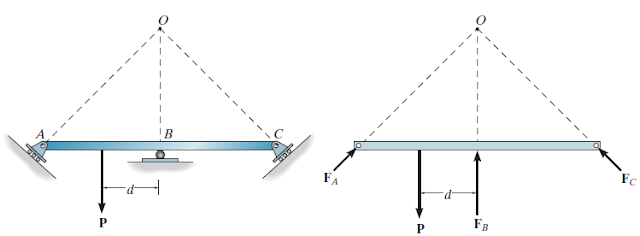### Stability of structures

satisfying equation of equilibrium doesn't mean that the structure is stable, aside from satisfying equilibrium equations, members shall be held and constrained by their supports to prevent these members from moving which can lead to collapsing of the structure. there is two cases in which proper constraining are not met.

partial constraints, in partial, constrain the structure reactions will be slightly fewer than the equations of equilibrium that must satisfy, in this case, the structure will partially constraints, figure 1 showing an example of a partially constrained structure. if we draw a free-body diagram for the L shape member, we can notice that the force p is inclined, therefore it will have x and y component. if we sum force in x-direction ΣFx=0, this equation will not be valid because the support will not constrain the member in the X direction, therefore our equilibrium equations are not satisfied.Figure 1

Improper constraints: in some case, the reactions will be equal to equilibrium equations but the structure is unstable, this will occur because the support is not constraining the structure properly. this can happen if the reactions at support concurrent at one point only. figure 2 showing an example of an unstable structure, reactions from supports are concurrent at point O, if we sum the moment at point o the result will not be zero, therefore the structure is unstable. also if all reactions are parallel then the structure will not be stable as shown in figure 3, for the structure in figure 3 the x-component of the force P will cause the structure to move.Figure 2Figure 3 Stability of a structure should be considered during the design stage, the unstable structure will move and collapse. if a structure consists of several numbers of members, each member should be checked for stability, it doesn't matter if the structure is determinate or indeterminate if it is unstable it will fail.